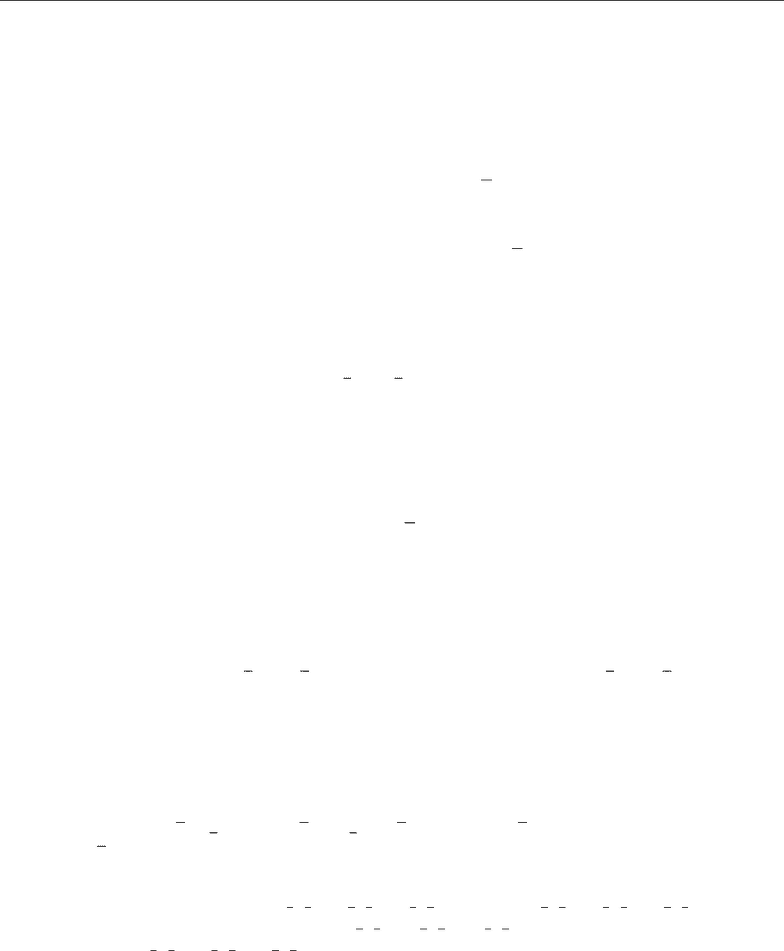# MATH 320 Chapter Notes - Chapter 3: Limit Point, Nested Intervals, Open Set

26 views3 pages
School
Department
Course
Professor

For unlimited access to Textbook Notes, a Class+ subscription is required.Orest Byskosh Homework 5 Due Monday October 23
Math 320-1, Fall 2017
1. Exercise 3.2.2
(a) Objective is to ﬁnd the limit points of the sets Aand B.
Recall the deﬁnition, a number a is limit point of set S if there exists a neighborhood of a
that contains an inﬁnite number of members of the set S.
xn= (1)n+2
n
then it follows that :
lim
n→∞
xn= lim{(1)n+2
n}
this equates to 1 if ais even and -1 if ais oﬀ, this fact stems from using the density of Q.
Therefore, 1 and -1 are the limit points of set A.
For every x[0,1], > 0,V(x) = (x, x +)B6=, which has inﬁnitely many rational
numbers all of which are limit points of B.
(b) Any point in A has the form 1 + 2
n,1 + 2
n. Take 1A, for every  > 0,V(1) 6⊂ A. Since 1
is not contained within the set, the set is not closed. Observe there is no open -neighborhood
of 1A, therefore Ais not open or closed.
B[0,1],[0,1] 6⊂ B, (x, x +)B6=.V(x) = (x, x +)6⊂ Band thus we see
that Bis not open or closed.
(c)
{(1)n+2
n:n= 1,2,3, ...}
From this we see that most of Aare isolated points, except for 1.
B[0,1] Bhas no isolated points.
(d) A= [A∪ { limit points of A}]=[A∪ {−1}]and B= [0,1].
2. Exercise 3.2.6
(a) False, Q(−∞,2) (2,)which are open sets; but, (−∞,2) (2,)6=R.
(b) The nested interval property is false for closed sets.
In= [n, )which is bounded and compact. Then I1I2... but In=.
(c) Let V6=be open and xV. Then, there exists an -neighborhood (x, x +)such that
(x, x +)VSince (x, x +)Vcontains inﬁnitely many rational numbers, so it
follows that every nonempty open set has rational numbers making the statement True.
(d) Let xn=3 + 1
n, V ={3}∪{1
n+3 : nN}.3is the only limit point of Vand
3V. Therefore, Vis closed, also Vdoes not contain and rational numbers making the
claim False.
(e) Cantor Set C= [0,1] [(1
3,2
3)(1
9,2
9)(7
9,8
9)...]with [(1
3,2
3)(1
9,2
9)(7
9,8
9)...]being
open. But, if follows than that [(1
3,2
3)(1
9,2
9)(7
9,8
9)...]cis closed. Therefore, C=
[0,1] [(1
3,2
3)(1
9,2
9)(7
9,8
9)...]cis closed, making the statement true.
3. Exercise 3.2.7
Unlock document

This preview shows page 1 of the document.
Unlock all 3 pages and 3 million more documents.

Already have an account? Log in

# Get access

Grade+
\$10 USD/m
Billed \$120 USD annually
Homework Help
Class Notes
Textbook Notes
40 Verified Answers
Study Guides
1 Booster Class
Class+
\$8 USD/m
Billed \$96 USD annually
Homework Help
Class Notes
Textbook Notes
30 Verified Answers
Study Guides
1 Booster Class